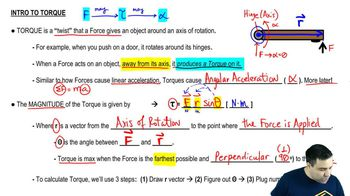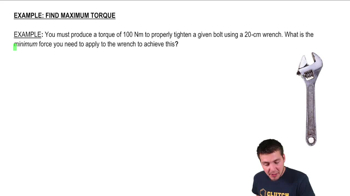Start typing, then use the up and down arrows to select an option from the list.
3:15 minutes
Problem 10a
Textbook Question

# A metal bar is in the xy-plane with one end of the bar at the origin. A force F = 97.00 N)i + (-3.00 N)j is applied to the bar at the point x = 3.00 m, y = 4.00 m. (b) What are the magnitude and direction of the torque with respect to the origin produced by F?Verified Solution
This video solution was recommended by our tutors as helpful for the problem above.
51views